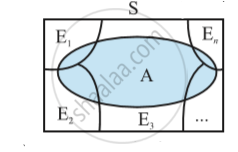HSC Science (Electronics) 11thMaharashtra State Board
Share

# Concept: Baye'S Theorem

#### description

• Partition of a sample space
• Theorem of total probability

#### notes

If E_1, E_2 ,..., E_n are n non empty events which constitute a partition of sample space S, i.e. E_1, E_2 ,..., E_n are pairwise disjoint and E_1 ∪ E_2 ∪ ... ∪ E_n = S and A is any event of nonzero probability, then

P(Ei|A) =(P(E_i) P (A | E_i))/(  sum_(i=1)^n P(E_j) P(A|E _j ))

P  for any i = 1, 2, 3, ..., n

Proof: By formula of conditional probability, we know that
P(E_i|A) = (P(A ∩ E_i )) / (P(A))
= (P(E_i ) (P(A|E_i )))/ (P(A)) (by multiplication rule of probability)

= (P(E_i )P(A|E_i ))/ (sum_(j = 1)^n P(E _j)P(A|E_j))  (by the result of theorem of total probability)

Remark:  The following terminology is generally used when Bayes' theorem is applied. The events E_1, E_2, ..., E_n are  called hypotheses.
The probability P(E_i) is called the priori probability of the hypothesis E_i
The conditional probability P(E_i |A) is called a posteriori probability of the hypothesis E_i.
Bayes' theorem is also called the formula for the probability of "causes". Since the E_i's are a partition of the sample space S, one and only one of the events E_i occurs (i.e. one of the events E_i must occur and only one can occur). Hence, the above formula gives us the probability of a particular Ei (i.e. a "Cause"), given that the event A has occurred.

1) Partition of a sample space:
A set of events E_1, E_2, ..., E_n is said to represent a partition of the sample space S if

(a) E_i ∩ E_j = φ, i ≠ j, i, j = 1, 2, 3, ..., n

(b) E_1 ∪ Ε_2 ∪ ... ∪ E_n= S   and

(c) P(E_i) > 0  "for all"   i = 1, 2, ..., n.
In other words, the events E_1, E_2, ..., E_n represent a partition of the sample space S if they are pairwise disjoint, exhaustive and have nonzero probabilities.
As an example, we see that any nonempty event E and its complement E′ form a partition of the sample space S since they satisfy E ∩ E′ = φ and E ∪ E′ = S.

2) Theorem of total probability:
Let   {E_1, E_2,...,E_n}  be a partition of the sample space S,  and suppose that each of the events E_1, E_2,..., E_n has nonzero probability of occurrence. Let A be any event associated with S, then
P(A) = P(E_1) P(A|E_1) + P(E_2) P(A|E_2) + ... + P(E_n) P(A|E_n)
=  sum _(j=1) ^ n P(E_j) P(A|E_j)

Proof :
Given that E_1, E_2,..., E_n is a partition of the sample space S in following fig.Therefore , S = E_1 ∪ E_2 ∪ ... ∪ E_n          ... (1)
and E_i ∩ E_j = φ, i ≠ j, i, j = 1, 2, ..., n
Now, we know that for any event A,
A = A ∩ S
= A ∩ (E_1 ∪ E_2 ∪ ... ∪ E_n)
= (A ∩ E_1) ∪ (A ∩ E_2) ∪ ...∪ (A ∩ E_n)
Also A ∩ E_i and A ∩ E_j are respectively the subsets of E_i and E_j. We know that E_i  and E_j are disjoint, for i  ≠ j, therefore, A  ∩ E_i and A  ∩ E_j  are also disjoint for all i ≠ j,  i, j = 1, 2, ..., n.
Thus,

P(A) = P [(A ∩ E_1) ∪ (A ∩ E_2)∪ .....∪ (A ∩ E_n)]

= P (A ∩ E_1) + P (A ∩ E_2) + ... + P (A ∩ E_n)
Now, by multiplication rule of probability, we have

P(A ∩ E_i) = P(E_i) P(A|E_i) as  P (E_i) ≠ 0 ∀ i = 1,2,..., n

Therefore, P (A) = P (E_1) P (A|E_1) + P (E_2) P (A|E_2) + ... + P (E_n)P(A|E_n)

or P(A) = sum_(j = 1)^n P(E_j) P(A|E_j)

Video list :https://youtu.be/_jY8B_0dZgo

### Shaalaa.com

probability part 02 [00:40:57]
S
1
0%

S